# Slope on a One to One Grid

On a one-to-one grid, we can count slope using the boxes. Slope# Review

The equation to calculate thecoordinate from thecoordinate on a line can be written in the form:…whereis the slope (also known as gradient or rate of change), andis the point on the line where the line cuts theaxis, that is, theintercept.

When given two points, we can calculate slope using the formula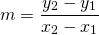Once we know the slope, we can calculate the valueby substituting one point in for theand; or we can use the formulaThe intercepts are points on the axes. To calculate theintercept, we substitute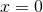to the equation of the line. To calculate theintercept, we substitute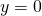to the equation of the line.

Vertical lines have an equation such as.

Horizontal lines have an equation such as.

Parallel lines have the same slope. E.g.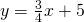andare parallel.

Perpendicular lines are lines that intersect at 90°. If the first line has slopethen the second line will have slope: the negative reciprocal. Eg, the linesand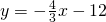are perpendicular. As another example, the lines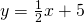andare perpendicular.

# Practice

Use this applet to practice calculating the slope between two points; finding the equation of a line; calculating the y-intercept and calculating the x-intercept.